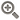﻿ 股市短线选股指标公式-点掌财经
• 综合
• 股票
• 板块
• 砖家
• 课程
• 说说
• 资讯

# 股市短线选股指标公式LC:=REF(CLOSE,1);

RSI5:=((SMA(MAX((CLOSE - LC),0),5,1) / SMA(ABS((CLOSE - LC)),5,1)) * 100);

TR1:=SUM(MAX(MAX((HIGH - LOW),ABS((HIGH - REF(CLOSE,1)))),ABS((LOW - REF(CLOSE,1)))),10);

HD:=(HIGH - REF(HIGH,1));

LD:=(REF(LOW,1) - LOW);

DMP:=SUM(IF(((HD > 0) AND (HD > LD)),HD,0),10);

DMM:=SUM(IF(((LD > 0) AND (LD > HD)),LD,0),10);

PDI:=((DMP * 100) / TR1);

MDI:=((DMM * 100) / TR1);

ADX:=MA(((ABS((MDI - PDI)) / (MDI + PDI)) * 100),5);

WR10:=((100 * (HHV(HIGH,10) - CLOSE)) / (HHV(HIGH,10) - LLV(LOW,10)));

NEWVOL:=(RSI5 - WR10);

股票最佳买入点:=(AV + NEWVOL);

最佳买入选股:=IF(CROSS(最佳买入,0),1,0);

VAR1:=SMA(最佳买入选股,3,1);

VAR2:=SMA(VAR1,3,1);

VAR3:=SMA(VAR2,3,1);

短线买点:IF(CROSS(VAR2,VAR3) AND (VAR2<40),5,0* 10000);

短线选股技巧：

1、看盘的首要重点是看板块和热点个股的轮动规律，进而推测出行情的大小和持续性时间变化。

比如每天应该注意是否有涨停个股开盘，如果有，那么说明主力资金还在努力选择突破口，如果两市都有10只以上的涨停个股开盘，则说明市场处于多头气氛，人气比较旺，少于这个标准则说明市场人气不佳，投资者应该当心大盘继续下跌风险。2、看盘应该注重关注成交量。

根据两市目前市值情况看，上海大盘成交量小于1000亿应做震荡整理理解，700亿以下为缩量，小于500亿可以理解为地量，超过1100亿应该理解为放量。地量背后往往意味着反转，例如，2010年6月底和7月初之间，先后多个交易日上海股市成交量低于500亿，这个时候空仓资金应为自己的重新进场做好准备。

3、努力培养盘感，运用技术手段捕捉市场机会。

不管是什么品种的股票，如经过短期暴跌，跌幅超过50%，下跌垂直度越大，那么关注价值就越高，当某一天突然缩量，短线买进的机会来了。因为急跌暴跌后，成交量突然萎缩就杀跌盘已经枯竭，肯定会出现反弹，这个时候可以坚决地战胜自己恐慌情绪积极进去抢一把反弹就走人。获取更多短线选股技巧就收藏本网。

#### 最新发布• 手机号码：

请输入手机号码，您的个人信息严格保密，请放心

• 图形验证码：

请输入右侧图形验证码

• 短信验证码：

请输入接收的短信验证码

获取短信验证码
X• 10牛
• 30牛
• 50牛
• 其它